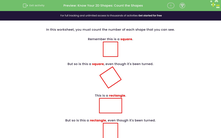# Know Your 2D Shapes: Count the Shapes

In this worksheet, students count the number of each shape that they can see.Key stage:  KS 1

Curriculum topic:   Geometry: Properties of Shapes

Curriculum subtopic:   Recognise 2D and 3D Shapes

Difficulty level:#### Worksheet Overview

In this worksheet, you must count the number of each shape that you can see.

Remember this is a square.But so is this a square, even though it's been turned.This is a rectangle.But so is this a rectangle, even though it's been turned.This is a triangle.This is a circle.Example

Count the circles.